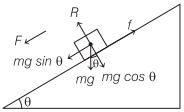# Motion on a Rough Inclined Plane | Definition, Example – Laws of Motion

## Motion on a Rough Inclined Plane | Definition, Example – Laws of Motion

We are giving a detailed and clear sheet on all Physics Notes that are very useful to understand the Basic Physics Concepts.

Motion of a body on a Rough Inclined Plane
When an object moves along an inclined plane then different forces act on it like normal reaction of plane, friction force acting in opposite direction of motion etc.

Different relations for the motion are given below
Normal reaction of plane, R = mg cos θ and net force acting downward on the block, F = mg sin θ – fAcceleration on inclined plane, a = g(sin θ – μ cos θ)
When angle of inclination of the plane from horizontal is less than the angle of repose (α), then
(i) minimum force required to move the body up the inclined plane

F1 = mg (sin θ + μ cos θ)

(ii) minimum force required to push the body down the inclined plane

F2 = mg (μ cos θ – sin θ)

Laws of Motion:
There are various laws in Physics that define the motion of the object. When an object is in motion whether it is linear or circular there is some force which is always imposed on it.